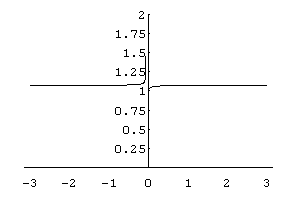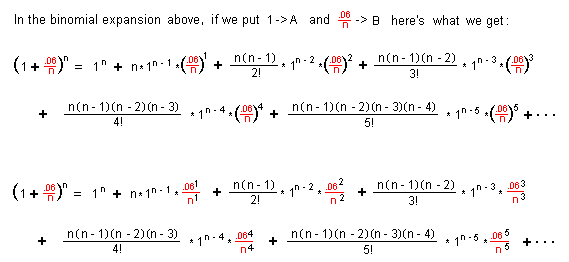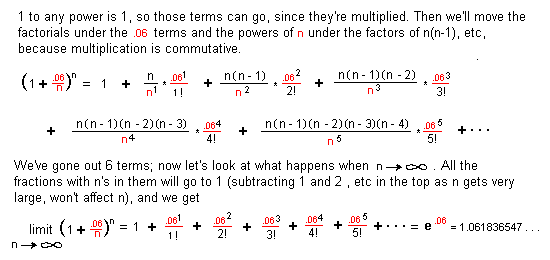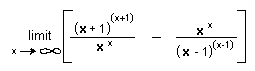## Answers for Chapter 11 Sample Problems

4. Compound interest
What would the amount you have in the bank after 1 year, putting in \$1, at 6%, compounded quarterly (4 times per year)?(1 + .06/4)4 = \$1.061363551
What would the amount you have in the bank after 1 year, putting in \$1, at 6%, compounded monthly (12 times per year)?(1 + .06/12)12 = \$1.061677812
What would the amount you have in the bank after 1 year, putting in \$1, at 6%, compounded daily (365 times per year)?(1 + .06/365)365 = \$1.061831311
What would the amount you have in the bank after 1 year, putting in \$1, at 6%, compounded 10,0000 times per year?(1 + .06/10,000)10,000 = \$1.061836355
What would the amount you have in the bank after 1 year, putting in \$1, at 6%, compounded continuously, (an infinite number of times per year)?
We end up with an infinite sequence
1.12, 1.061363551, 1.061677812, 1.061831311, 1.061836355, 1.0618363547... which approaches the limitn->inf(1 + .06/n)n = 1.0618363547... = e.06 WOW!

Kirsten, now 15, started with me at age 5. On Sept. 10, 1996 she was working on this problem (using .07 instead of .06) for her calculus class. We graphed (1 + .07/x)x in Derive, but couldn't import it into a paint program and then to a gif file. So we graphed it in Mathematica and got this:Here are some of the things we explored in Mathematica and Derive with some surprising and exciting results:
(1 + .07/-0.1)-0.1 = 1.12794, a real number. Check the graph.
(1 + .07/0)0 = 1 This was hard to believe, but the graph bears it out!
(1 + .07/0.001)0.001 = 1.00427
(1 + .07/-0.07)-0.07 Mathematica gives: "Power::infy: Infinite expression 0.-0.07 encountered. ComplexInfinity." as the answer. Derive gives " + or - infinity" as the answer.
(1 + .07/-0.01)-0.01 = 0.981757 - 0.030853i ; which makes sense when you look at the graph.

limitx->0(1 + .07/x)x = 1
limitx->0,Direction->-0(1 + .07/x)x Mathematica gives: "Power::infy: Infinite expression 1/0 encountered. Infinity::indet: Indeterminate expression ComplexInfinity" 0 encountered.
Indeterminate"

limitx->infinity(1 + .07/x)x = 1.072508181254216 and e.07 = 1.072508181254216
The first 6 terms of the binomial expansion are:9. Ian looked for patterns always, and found= e .